# Android—以面试角度剖析HashMap源码

## 前言

HashMap 这个词想必大家都挺熟悉的！但往往大多数都知其所用，而不知其原理，导致面试的处处碰壁！因此，这一篇的作用就是以面试的角度剖析HashMap！话不多说，直接开始！

## 1、为什么要学HashMap?

• HashMap的原理？内部数据结构？
• HashMap中put方法的过程是怎样实现的？
• HashMap中hash函数是怎样实现的？
• HashMap是怎样扩容的呢？
• HashMap中某个Entry链太长，查找时间复杂度可能达到O(n)，怎么优化？

## 2、剖析HashMap

### 2.1 HashMap初始化

``````HashMap<Integer,Integer> hashMap = new HashMap<>(6, 1);
HashMap<Integer, Integer> hashMap2 = new HashMap<>();

``````
/**
* The default initial capacity - MUST be a power of two.
*/
static final int DEFAULT_INITIAL_CAPACITY = 1 << 4; // aka 16

/**
* The load factor used when none specified in constructor.
*/
static final float DEFAULT_LOAD_FACTOR = 0.75f;

public HashMap() {
this.loadFactor = DEFAULT_LOAD_FACTOR; // all other fields defaulted
}

1. 初始化给`this.loadFactor`赋值为`0.75f`

2. 而这个0.75f就是该HashMap对应的扩展因子。（扩展因子：当长度大于等于 容量长度*扩展因子时，需要对该map进行扩容）

3. 而 map默认长度就是`DEFAULT_INITIAL_CAPACITY=1 << 4`也就是默认16

4. 结合扩展因子一起看，也就是说，当map长度大于等于 16*0.75f的时候，对应map需要扩容！（至于怎么扩容，下面会讲解）

``````public HashMap(int initialCapacity, float loadFactor) {
if (initialCapacity < 0)
throw new IllegalArgumentException("Illegal initial capacity: " +
initialCapacity);
if (initialCapacity > MAXIMUM_CAPACITY)
initialCapacity = MAXIMUM_CAPACITY;
throw new IllegalArgumentException("Illegal load factor: " +
this.threshold = tableSizeFor(initialCapacity);
}

1. `this.loadFactor = loadFactor`这个在上面讲过，就是给扩展因子赋值，只不过由默认变成了手动
2. `this.threshold = tableSizeFor(initialCapacity);` 这里我们看到调用了`tableSizeFor`方法，并将入参一带入该方法中！

#### 2.1.1 tableSizeFor 方法剖析

``````
/**
* The maximum capacity, used if a higher value is implicitly specified
* by either of the constructors with arguments.
* MUST be a power of two <= 1<<30.
*/
static final int MAXIMUM_CAPACITY = 1 << 30;

/**
* Returns a power of two size for the given target capacity.
*/
static final int tableSizeFor(int cap) {
int n = cap - 1;
n |= n >>> 1;
n |= n >>> 2;
n |= n >>> 4;
n |= n >>> 8;
n |= n >>> 16;
return (n < 0) ? 1 : (n >= MAXIMUM_CAPACITY) ? MAXIMUM_CAPACITY : n + 1;
}

1. `>>>`表示无符号右移，也叫逻辑右移，即若该数为正，则高位补0，而若该数为负数，则右移后高位同样补0。 按二进制形式把所有的数字向右移动对应的位数，低位移出（舍弃），高位的空位补零。对于正数来说和带符号右移相同，对于负数来说不同。其他结构和>>相似。

2. `|`表示的是或运算，即两个二进制数同位中，只要有一个为1则结果为1，若两个都为1其结果也为1，换句话说就是取并集。

1. `int n = cap - 1` 这个时候 `n=5`

2. `n |= n >>> 1` 这个时候需要将这句代码拆成两部解析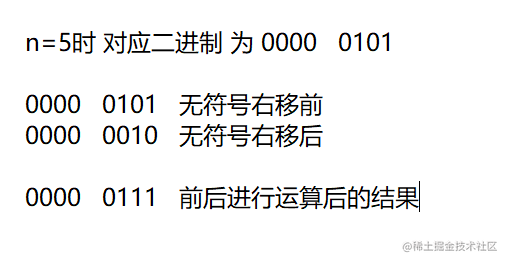1. 继续往下走当执行`n |= n >>> 2`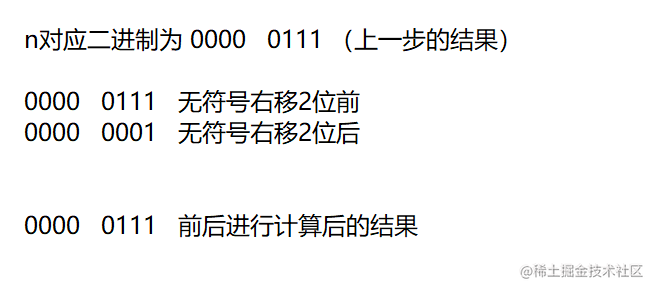1. 此时不管是`n >>> 4` 还是`n >>> 16` 因为是取并集结果都为 `0000 0111` 转为十进制为 `n=7`
2. 那么看看最后一句`(n < 0) ? 1 : (n >= MAXIMUM_CAPACITY) ? MAXIMUM_CAPACITY : n + 1;` 最终结果为`n=8`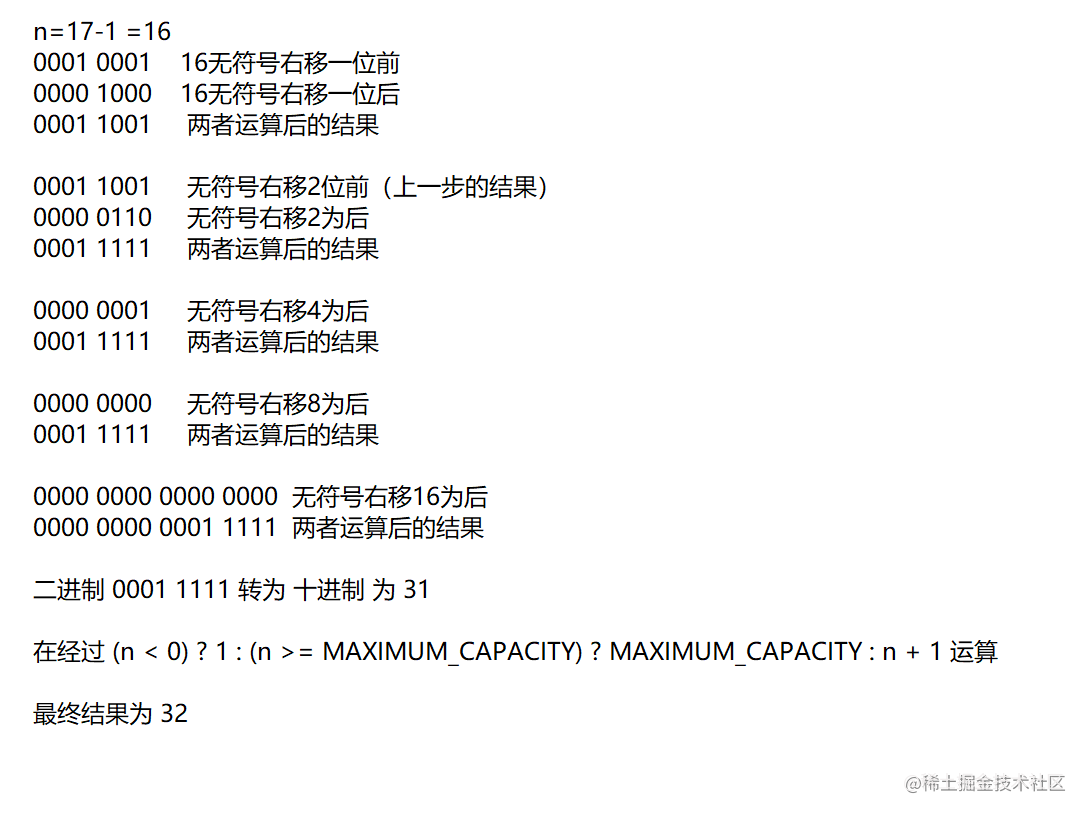OK！到这HashMap的初始化已经剖析完成了。接下来该剖析HashMap的put操作！

### 2.2 HashMap对应put操作

``````public V put(K key, V value) {
return putVal(hash(key), key, value, false, true);
}

#### 2.2.1 HashMap对应Hash算法

``````static final int hash(Object key) {
int h;
return (key == null) ? 0 : (h = key.hashCode()) ^ (h >>> 16);
}

`````` 0000 0000 0001 0001
0000 0000 0001 0111
——————————
0000 0000 0000 0110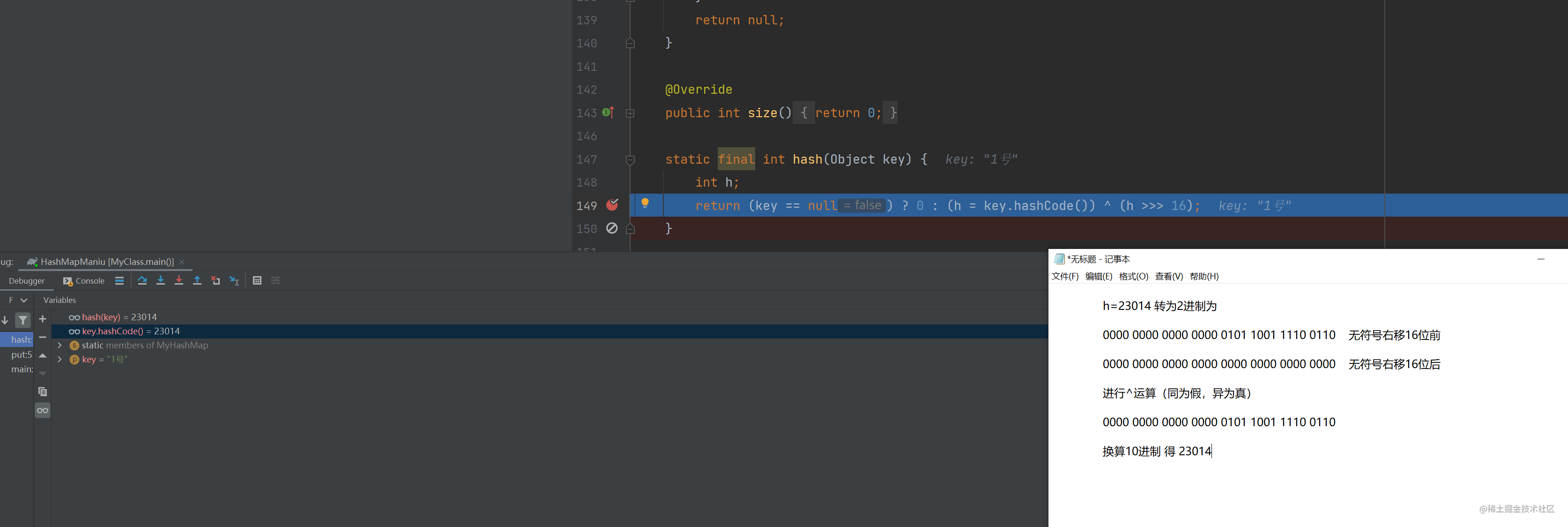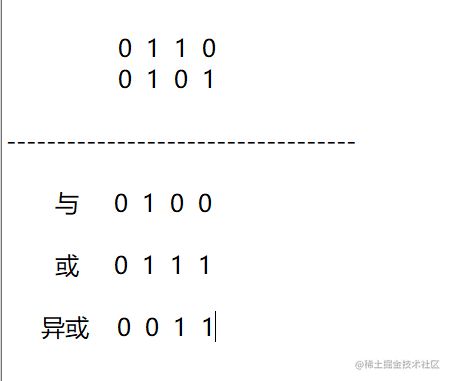• 或运算：（只要有1，那么就是1） 0|0=0 ，1|0=1 ，0|1=1 ，1|1=1 我们看到有三者都为1
• 与运算：（都是1时，结果才为1） 0&0=0 ，0&1=0 ，1&0=0 ，1&1=1 我们看到有三者都为0
• 异或预算：（只要一样结果就是0）0^0=0 ，0^1=1 ，1^0=1 ，1^1=0 我们看到有两者为0，两者为1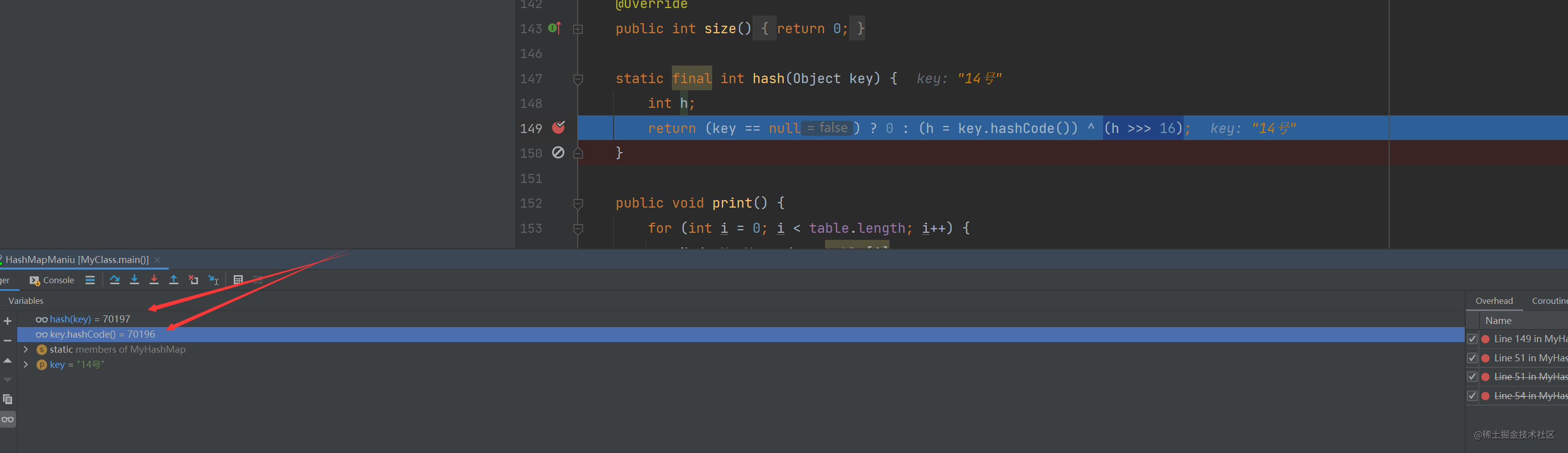#### 2.2.2 HashMap内部构造结构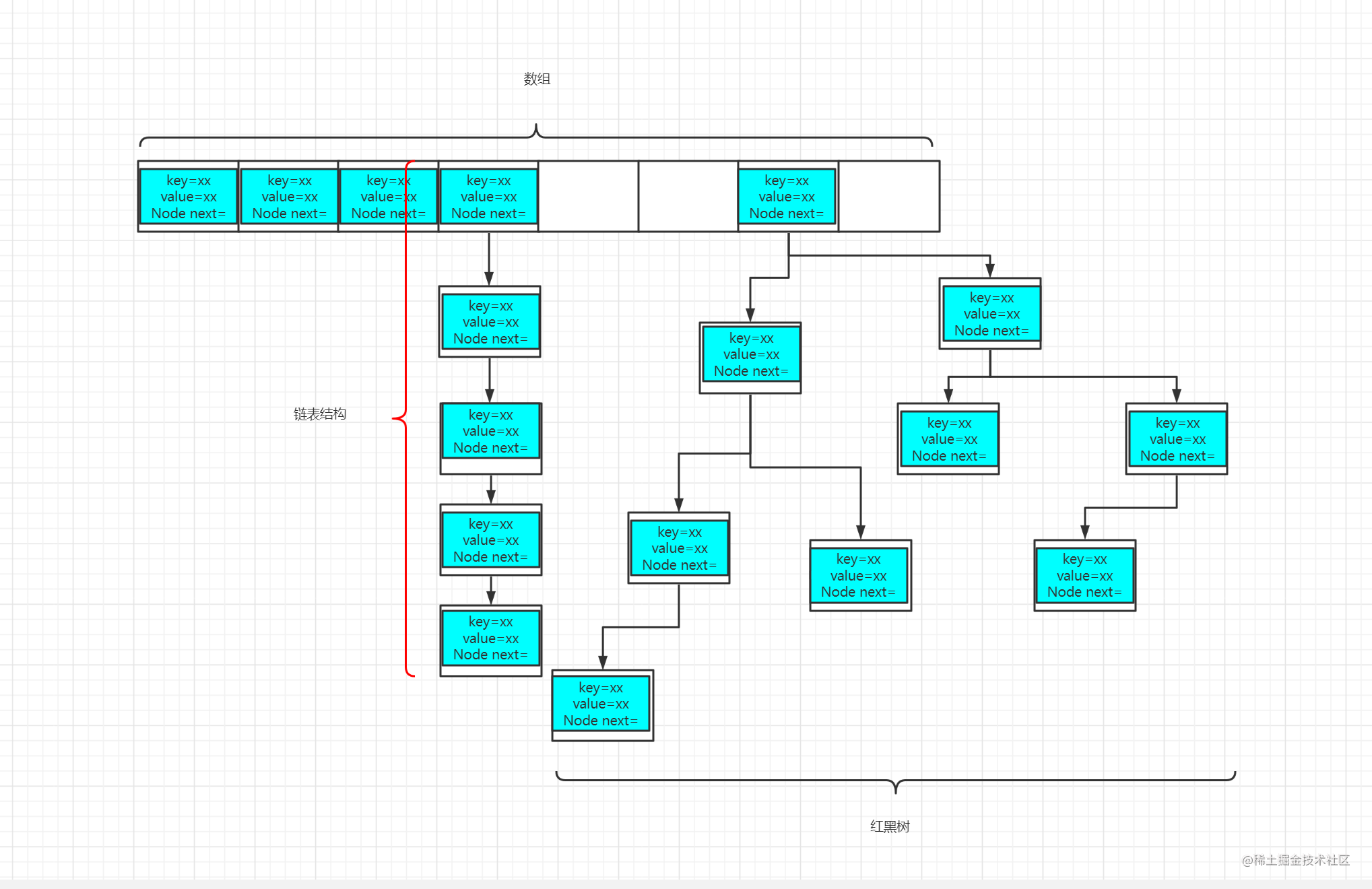• HashMap内部构造为数组+链表的形式，而数组的默认长度要么是标准的16，要么就是`tableSizeFor`方法返回的结果
• 当链表长度大于等于8，并且数组长度大于64时，将会转为红黑树结构

1. 因为它是数组结构，所以第一时间得要找到能存储该值的下标，只有找到对应下标了才能更好的保存值
2. 找到对应下标了，再看该下标是否存在链表结构，如果不存在则创建新的链表结构，并将对应key-value存储起来
3. 如果存在对应链表结构，则判断该链表是否转化为红黑树，如果真，则按红黑树原理存储或者替换对应值
4. 如果非红黑树结构，则判断对应key是否在该链表中，如果在链表中，则直接替换原有值
5. 如果对应key不存在原有链表中，则先判断该链表长度是否大于等于7，如果真，则创建新的单元格按红黑树的原理存储对应元素，最终长度自增1位；（因为长度满足8位就是红黑树结构，因此要在自增前判断是否满足要求）
6. 如果链表长度小于7，那么创建新的单元格直接存入该链表中，并与上一个单元格next相互关联

#### 2.2.3 putVal方法剖析

``````

/**
* The next size value at which to resize (capacity * load factor).
*
* @serial
*/

int threshold;

static final int TREEIFY_THRESHOLD = 8;

/**
* Implements Map.put and related methods
*
* @param hash hash for key
* @param key the key
* @param value the value to put
* @param onlyIfAbsent if true, don't change existing value
* @param evict if false, the table is in creation mode.
* @return previous value, or null if none
*/
final V putVal(int hash, K key, V value, boolean onlyIfAbsent,
boolean evict) {
Node<K,V>[] tab; Node<K,V> p; int n, i;
if ((tab = table) == null || (n = tab.length) == 0)
n = (tab = resize()).length;  //分析点1

if ((p = tab[i = (n - 1) & hash]) == null)  //分析点2

tab[i] = newNode(hash, key, value, null);
else {
Node<K,V> e; K k;
if (p.hash == hash &&
((k = p.key) == key || (key != null && key.equals(k))))
e = p; //分析点3

else if (p instanceof TreeNode) //分析点 4
e = ((TreeNode<K,V>)p).putTreeVal(this, tab, hash, key, value);

else {

for (int binCount = 0; ; ++binCount) {
if ((e = p.next) == null) {
p.next = newNode(hash, key, value, null);  // 分析点5

if (binCount >= TREEIFY_THRESHOLD - 1) // -1 for 1st
treeifyBin(tab, hash);    // 分析点5-1

break;
}
if (e.hash == hash &&
((k = e.key) == key || (key != null && key.equals(k))))
break;  //分析点6

p = e; //分析点7

}
}
if (e != null) { // existing mapping for key
V oldValue = e.value;
if (!onlyIfAbsent || oldValue == null)  //分析点8
e.value = value;
afterNodeAccess(e);
return oldValue;
}
}
++modCount;
if (++size > threshold)
resize(); //分析点9
afterNodeInsertion(evict);
return null;
}

• 分析点1：当该map第一次进行put操作时，对应的tab数组并未初始化。因此这里需要调用`resize()`方法，并给变量n赋值（分析点9会单独讲解该方法

• 分析点2：这里使用了`(p = tab[i = (n - 1) & hash]) == null`这句代码，从该方法前两句可以看出

• n表示该HashMap对应数组长度
• tab表示该HashMap对应数组
• hash表示该方法的第一个入参，是由上一个方法根据hash算法推算出具有散列性的值
• `i = (n - 1) & hash` 这句代码就是通过 hash算法推算的值与数组长度-1进行运算，取出对应的下标，因为hash具有散列性（平均），因此能够均匀的分配对应数组单元格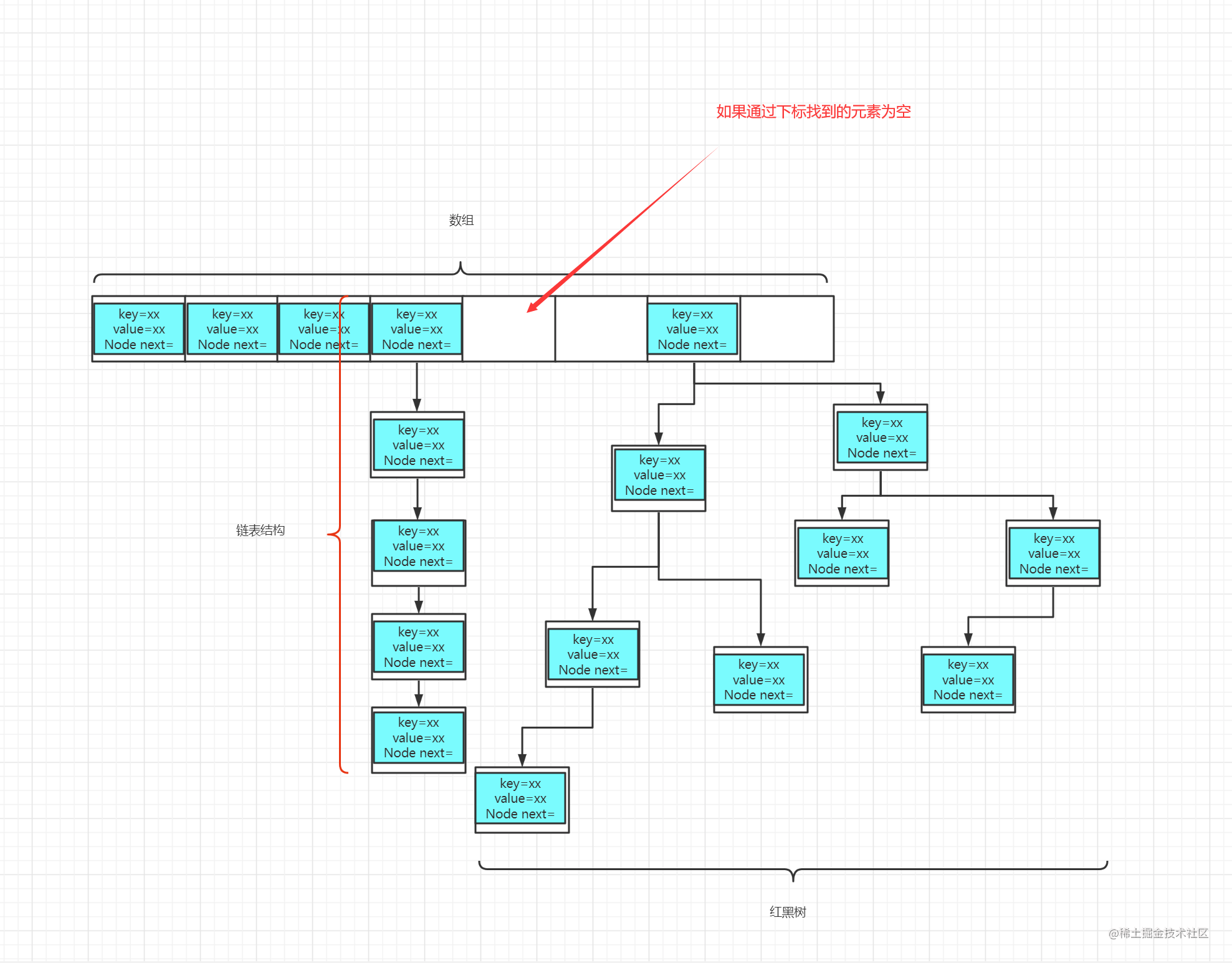• 如果通过下标找到元素为空，那么就创建新的链表结构，并将当前key-value存入对应链表结构中
• 分析点3：这里使用了`p.hash == hash && ((k = p.key) == key || (key != null && key.equals(k)))` 这句代码，结合分析点2一起看可以得出：

• p 表示通过下标找到的对应链表结构，并且非空
• k 表示该方法第二个入参，表示对应key值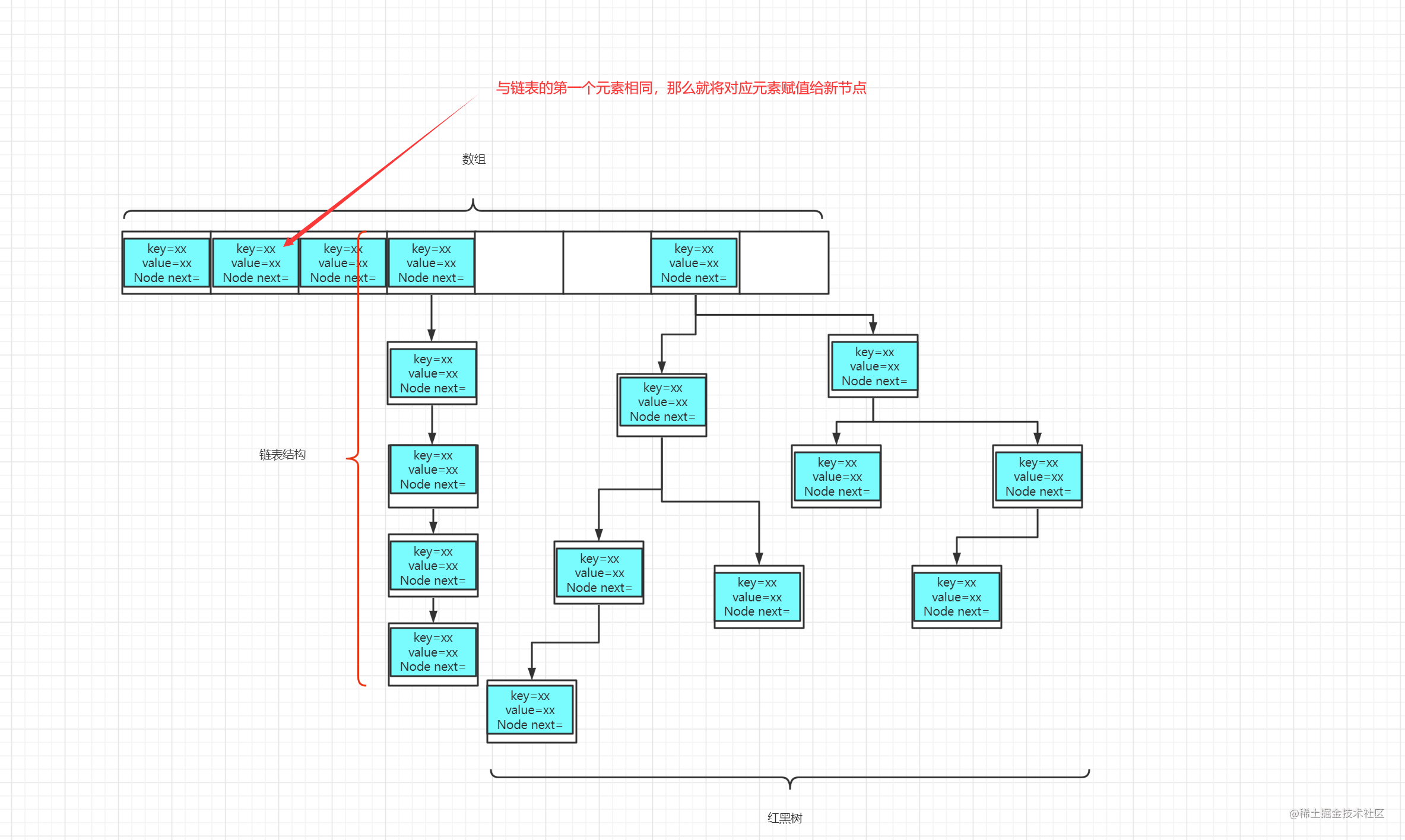• 因此该判断条件意思：如果输入的key，与链表第一个元素的key相同，那么将该单元格赋值给创建的`e`节点 （分析点8还会继续讲解该变量
• 分析点4：这里使用了`e = ((TreeNode<K,V>)p).putTreeVal(this, tab, hash, key, value)` 结合判断条件可以看出

• p 表示通过下标找到的对应链表结构，并且非空
• `p instanceof TreeNode` 这句判断条件表示，该链表结构是否 `TreeNode` 类型（红黑树结构）
• 这里红黑树就不详解了，结构组成总结起来就一句话：你比我大，那去这一边，比我小，那就去另外一边，一直往下每个节点都这样判断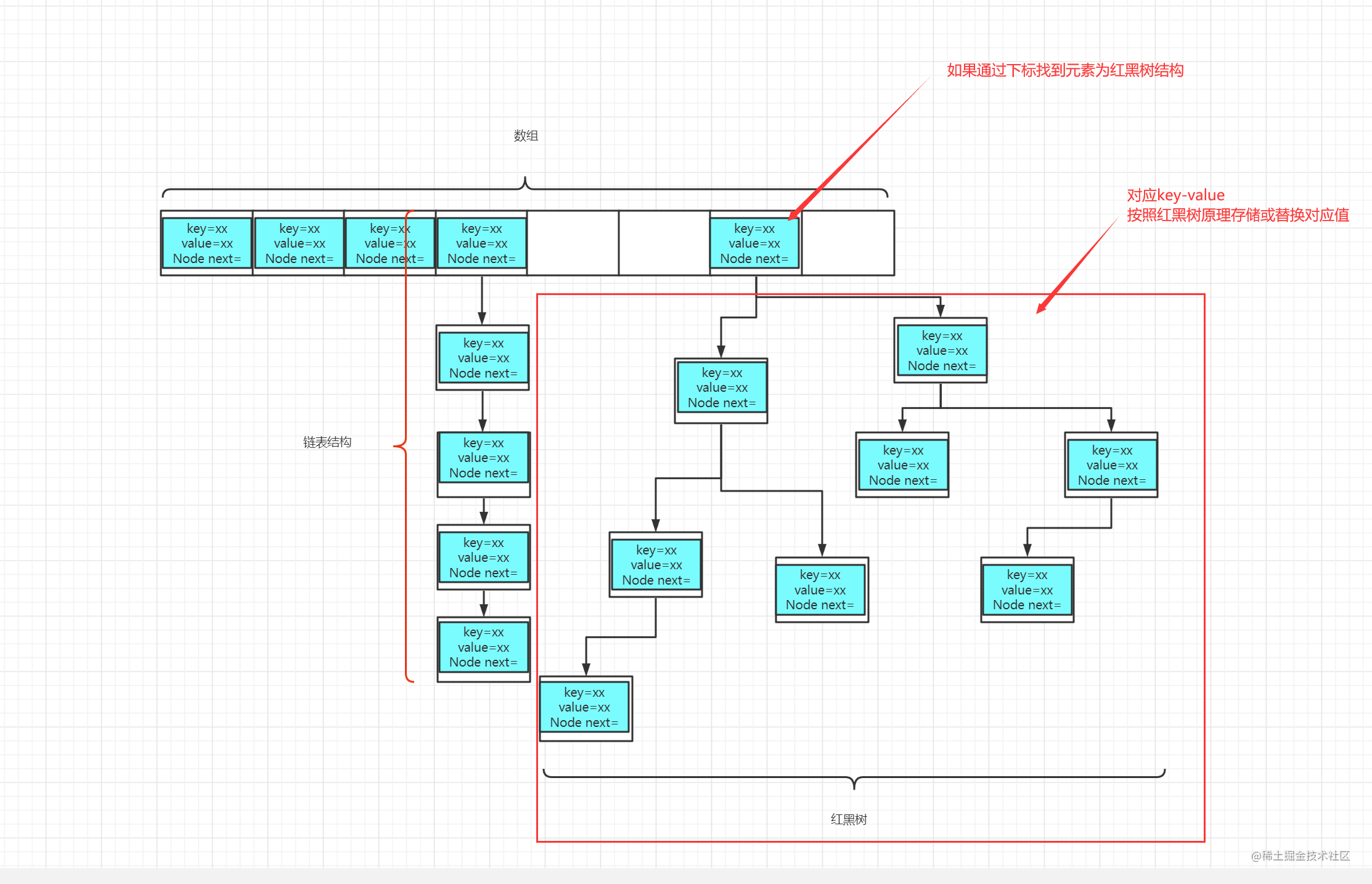• 因此这里具体意思就是：如果为红黑树结构，那就按照红黑树结构存储替换值，并且将对应节点返回赋值给上面创建的`e`节点（分析点8还会继续讲解该变量
• 分析点5：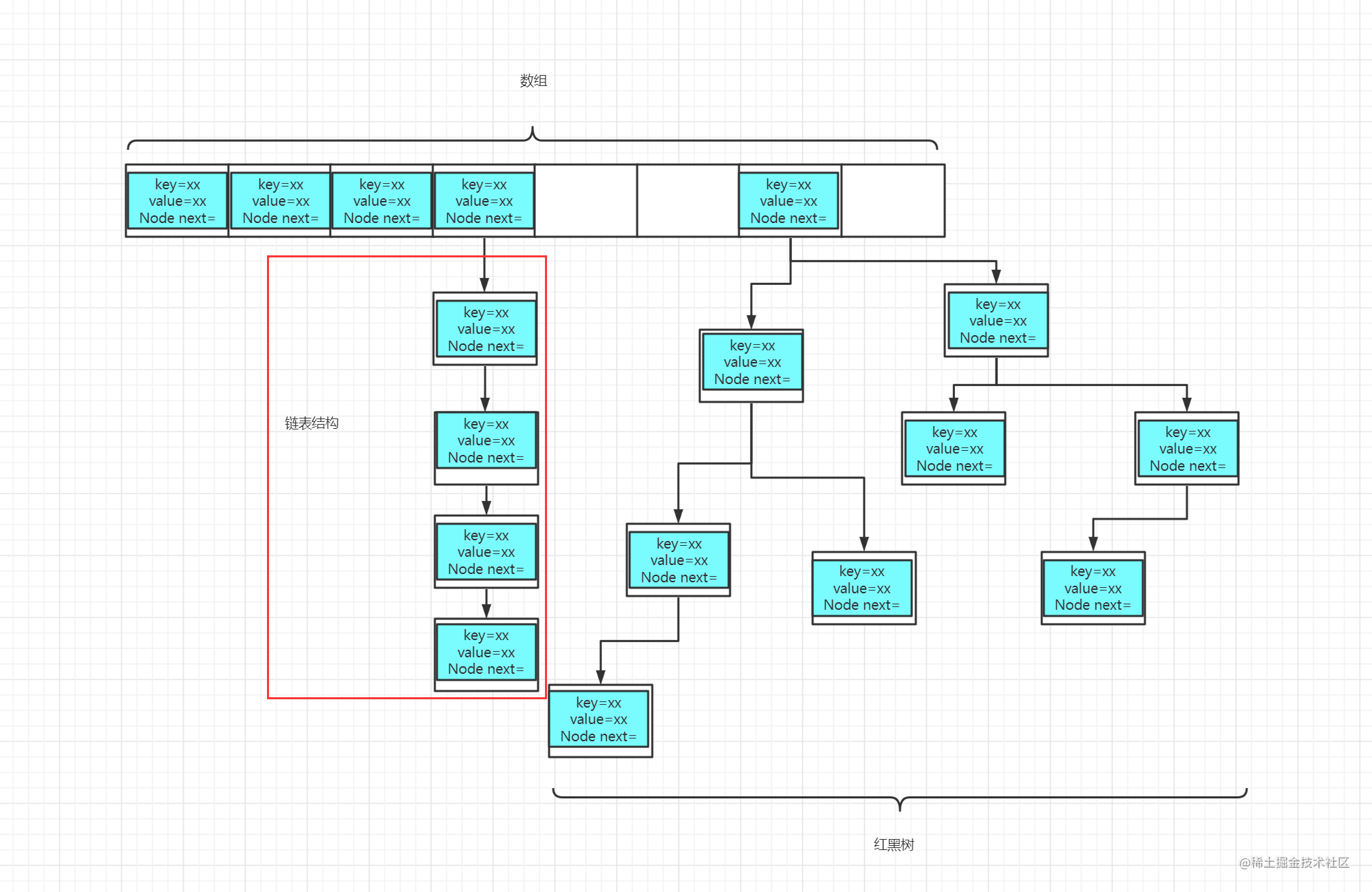• 逻辑执行此处，这说明已经不满足上述分析点，也就是说，处理的单位只会存在标注的红框里
• `(e = p.next) == null` 这句代码表示，如果往下找已经没有节点了，那么执行`p.next = newNode(hash, key, value, null)` 创建新的单元格并将对应key-value存储起来并与`p.next`相互关联
• 分析点5-1：

• 结合分析点5一起看，上一步将创建的单元格与`p.next`相关关联后
• `TREEIFY_THRESHOLD` 该变量=8
• `binCount >= TREEIFY_THRESHOLD - 1` 这句代码意思是，判断当前链表是否大于等于7 ，因为自增在下文，因此这里需要减一。
• `treeifyBin(tab, hash);` 这句代码意思是，满足上面判断条件，将会调用该方法！（之前没有详看该方法，以为这个方法就直接转红黑树了，没想到里面还有判断。感谢《sam码农提醒》）
``````
static final int MIN_TREEIFY_CAPACITY = 64;

final void treeifyBin(Node<K,V>[] tab, int hash) {
int n, index; Node<K,V> e;
if (tab == null || (n = tab.length) < MIN_TREEIFY_CAPACITY) //分析5-2
resize();
else if ((e = tab[index = (n - 1) & hash]) != null) {
TreeNode<K,V> hd = null, tl = null;
do {
TreeNode<K,V> p = replacementTreeNode(e, null);
if (tl == null)
hd = p;
else {
p.prev = tl;
tl.next = p;
}
tl = p;
} while ((e = e.next) != null);
if ((tab[index] = hd) != null)
hd.treeify(tab);
}
}
复制代码``````
• 分析5-2：这里我们可以看到：虽然进该方法满足了链表长度大于等于8，但是在5-2位置还额外判断了数组长度是否超过64，如果没超过，依然走的扩容。超过了才会转红黑树

• 分析点6：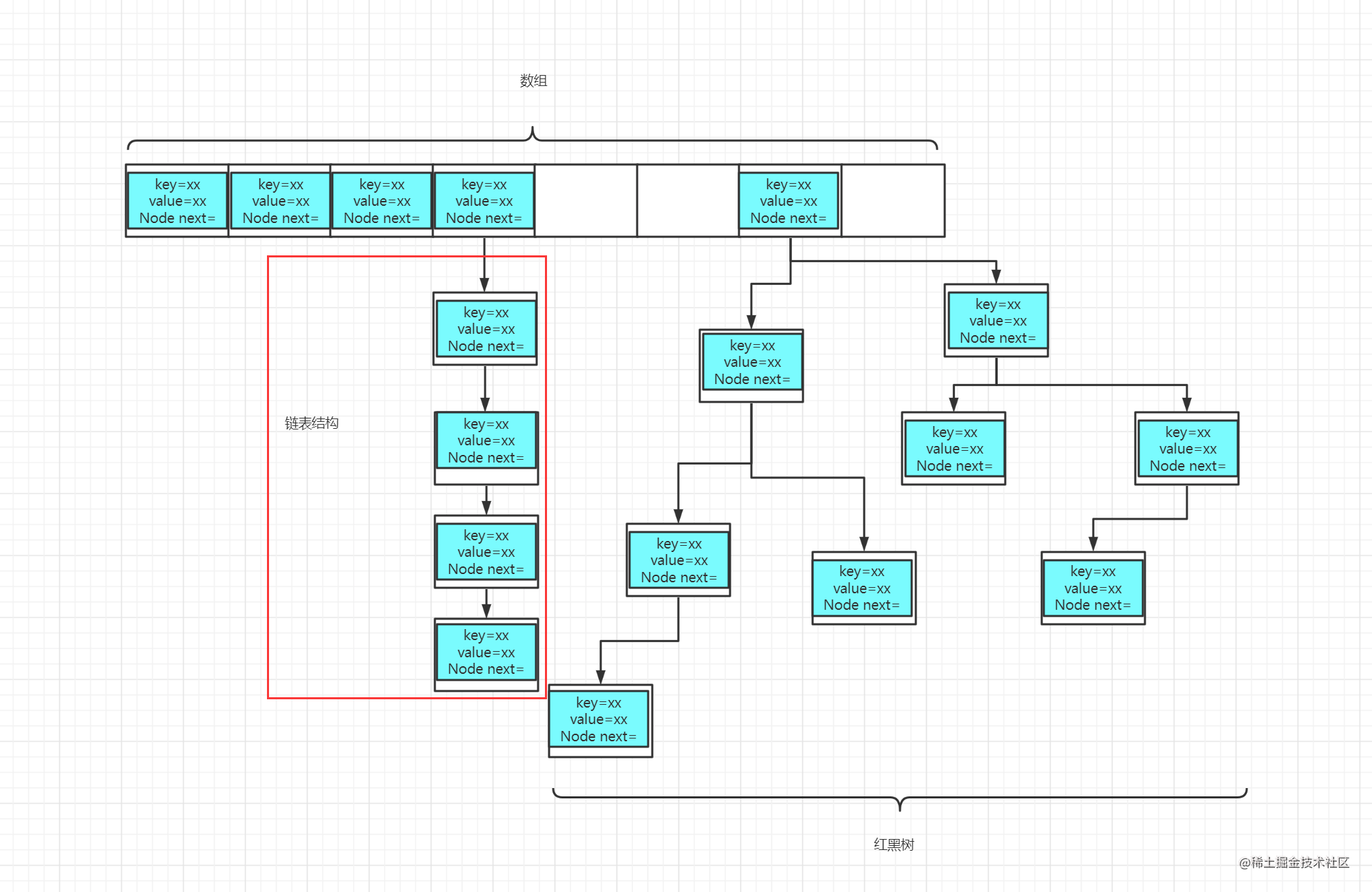• e 在分析5 执行了 `e = p.next` 并且不为null
• `(e.hash == hash && ((k = e.key) == key || (key != null && key.equals(k)))` 这句意思表示在红框标注里是否通过key找到了对应的单元格，如果真则跳出循环；如果假则执行分析7
• 分析点7：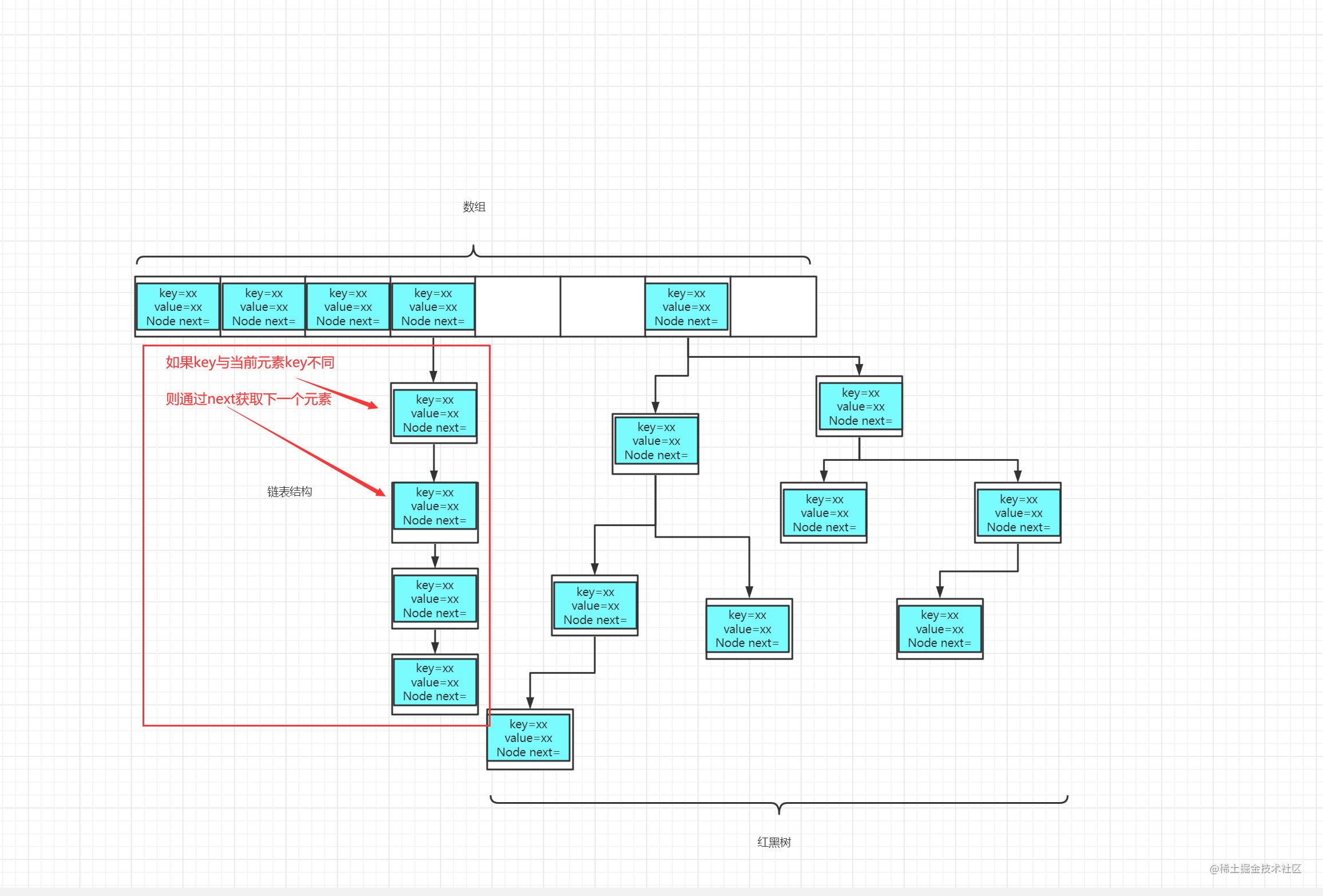• 结合分析6一起看，如果当前key与当前单元格对应key不等，那么就执行`p = e;` 指向下一个单元格
• 分析点8：

• `onlyIfAbsent` 该变量为方法的第4个入参，value=false

• 能进入该逻辑，因此对应`e`不为空！上述条件中，满足条件有：分析3、分析4、分析6

• 这三者条件都是满足对应key相同则赋值，那么这里就是替换对应相同key对应的value值

• 分析点9：

• 能进分析9，则说明满足`++size > threshold`条件
• size表示该map中所有key-value 的总长度
• threshold 表示 达到扩容条件的目标值
• `resize()`方法，那就是扩容了。那么这个方法到底做了甚么呢？
##### 2.2.3.1 扩容 `resize()` 方法

1. 分析点1 在table=null 或者table.length=0的时候会调用该方法
2. 分析点9 在满足`++size > threshold`条件时，会调用该方法

``````

static final float DEFAULT_LOAD_FACTOR = 0.75f;

static final int DEFAULT_INITIAL_CAPACITY = 1 << 4; // aka 16

final Node<K,V>[] resize() {
Node<K,V>[] oldTab = table;
int oldCap = (oldTab == null) ? 0 : oldTab.length;
int oldThr = threshold;
int newCap, newThr = 0;
if (oldCap > 0) {
.....
}
else if (oldThr > 0){

//初始化HashMap时，调用了有参构造器，就会进入该逻辑
newCap = oldThr;

}
else {
//初始化HashMap时，调用无参构造器，就会进入该逻辑
newCap = DEFAULT_INITIAL_CAPACITY;
newThr = (int)(DEFAULT_LOAD_FACTOR * DEFAULT_INITIAL_CAPACITY);
}
if (newThr == 0) {

//初始化HashMap时，调用了有参构造器，就会进入该逻辑
float ft = (float)newCap * loadFactor;
newThr = (newCap < MAXIMUM_CAPACITY && ft < (float)MAXIMUM_CAPACITY ?
(int)ft : Integer.MAX_VALUE);
}
threshold = newThr;
@SuppressWarnings({"rawtypes","unchecked"})
Node<K,V>[] newTab = (Node<K,V>[])new Node[newCap];
table = newTab;
.....
return newTab;
}

• 已知条件为：table =null , oldCap=0

• 默认情况下将会直接进入else 相关逻辑 ，如果用户初始化HashMap调用的有参构造器，那么就会执行代码注释标注的部分（下面所有都按初始化时，调用无参构造器讲解）

• 当执行`newCap = DEFAULT_INITIAL_CAPACITY` 对应newCap=16

• 当执行`(int)(DEFAULT_LOAD_FACTOR * DEFAULT_INITIAL_CAPACITY)`对应 newThr=16*0.75f

• 当执行`threshold = newThr` 对应threshold=12

• 当执行`Node<K,V>[] newTab = (Node<K,V>[])new Node[newCap]`以及`table = newTab` 对应table长度为`newCap`也就是默认16

``````static final float DEFAULT_LOAD_FACTOR = 0.75f;

static final int DEFAULT_INITIAL_CAPACITY = 1 << 4; // aka 16

static final int MAXIMUM_CAPACITY = 1 << 30;

final Node<K,V>[] resize() {
Node<K,V>[] oldTab = table;
int oldCap = (oldTab == null) ? 0 : oldTab.length;
int oldThr = threshold;
int newCap, newThr = 0;
if (oldCap > 0) {
if (oldCap >= MAXIMUM_CAPACITY) { //分析点10
threshold = Integer.MAX_VALUE;
return oldTab;
}
else if ((newCap = oldCap << 1) < MAXIMUM_CAPACITY &&
oldCap >= DEFAULT_INITIAL_CAPACITY)  //分析点11
newThr = oldThr << 1; // double threshold
}

//删除上面已经讲解过的代码.....

threshold = newThr;
@SuppressWarnings({"rawtypes","unchecked"})
Node<K,V>[] newTab = (Node<K,V>[])new Node[newCap]; //分析点12
table = newTab;
if (oldTab != null) {
for (int j = 0; j < oldCap; ++j) {
Node<K,V> e;
if ((e = oldTab[j]) != null) {
oldTab[j] = null;
if (e.next == null)
newTab[e.hash & (newCap - 1)] = e;
else if (e instanceof TreeNode)
((TreeNode<K,V>)e).split(this, newTab, j, oldCap);
else { // preserve order
Node<K,V> loHead = null, loTail = null;
Node<K,V> hiHead = null, hiTail = null;
Node<K,V> next;
do {
next = e.next;
if ((e.hash & oldCap) == 0) {
if (loTail == null)
else
loTail.next = e;
loTail = e;
}
else {
if (hiTail == null)
else
hiTail.next = e;
hiTail = e;
}
} while ((e = next) != null);
if (loTail != null) {
loTail.next = null;
}
if (hiTail != null) {
hiTail.next = null;
newTab[j + oldCap] = hiHead;
}
}
}
}
}
return newTab;
}

• 分析点10：这里是为了做最大限制扩容，如果扩容前的长度已经达到了 `1<<30` ，那么此次扩容长度将会是最大值`Integer.MAX_VALUE`

• 分析点11：我们来拆分一下这段条件判断代码：`(newCap=oldCap<<1<MAXIMUM_CAPACITY&&oldCap>=DEFAULT_INITIAL_CAPACITY`

• 执行`newCap=oldCap<<1` 时，对应 newCap=扩容前的长度<<1 ，也就是 16<<1 ，最终结果为 32

• 在判断逻辑里，当执行`newThr = oldThr << 1` 时，也就是 12<<1，最终结果为 24

• 分析点12：将分析11的结果创建了一个全新的数组，并在下面的循环中，将原有数组里的内容赋值给这个全新数组

### 2.3 HashMap对应get操作

``````public V get(Object key) {
Node<K,V> e;
return (e = getNode(hash(key), key)) == null ? null : e.value;
}

• 这里我们可以看到 依然调用了两个方法 `hash(xx)``getNode(xx,xx)`

• `hash(xx)`这个在put操作里讲解过，这里不再赘述

• 因此现在只需要讲解这个方法`getNode(xx,xx)` 即可

#### 2.3.1 getNode 方法剖析

``````final Node<K,V> getNode(int hash, Object key) {
Node<K,V>[] tab; Node<K,V> first, e; int n; K k;
if ((tab = table) != null && (n = tab.length) > 0 &&
(first = tab[(n - 1) & hash]) != null) { //分析点1

if (first.hash == hash && // always check first node
((k = first.key) == key || (key != null && key.equals(k))))
return first; //分析点2

if ((e = first.next) != null) {
//分析点3
if (first instanceof TreeNode)
return ((TreeNode<K,V>)first).getTreeNode(hash, key);
do {
if (e.hash == hash &&
((k = e.key) == key || (key != null && key.equals(k))))
return e;
} while ((e = e.next) != null);
}
}
return null; //分析点4
}

• 分析点1：这里仅仅是对map里面的数组进行判断，看是否为有效有值的数组，并将有效值给first赋值

• 分析点2：这里表示如果是在第一层节点通过key找到对应节点时，那就直接返回对应节点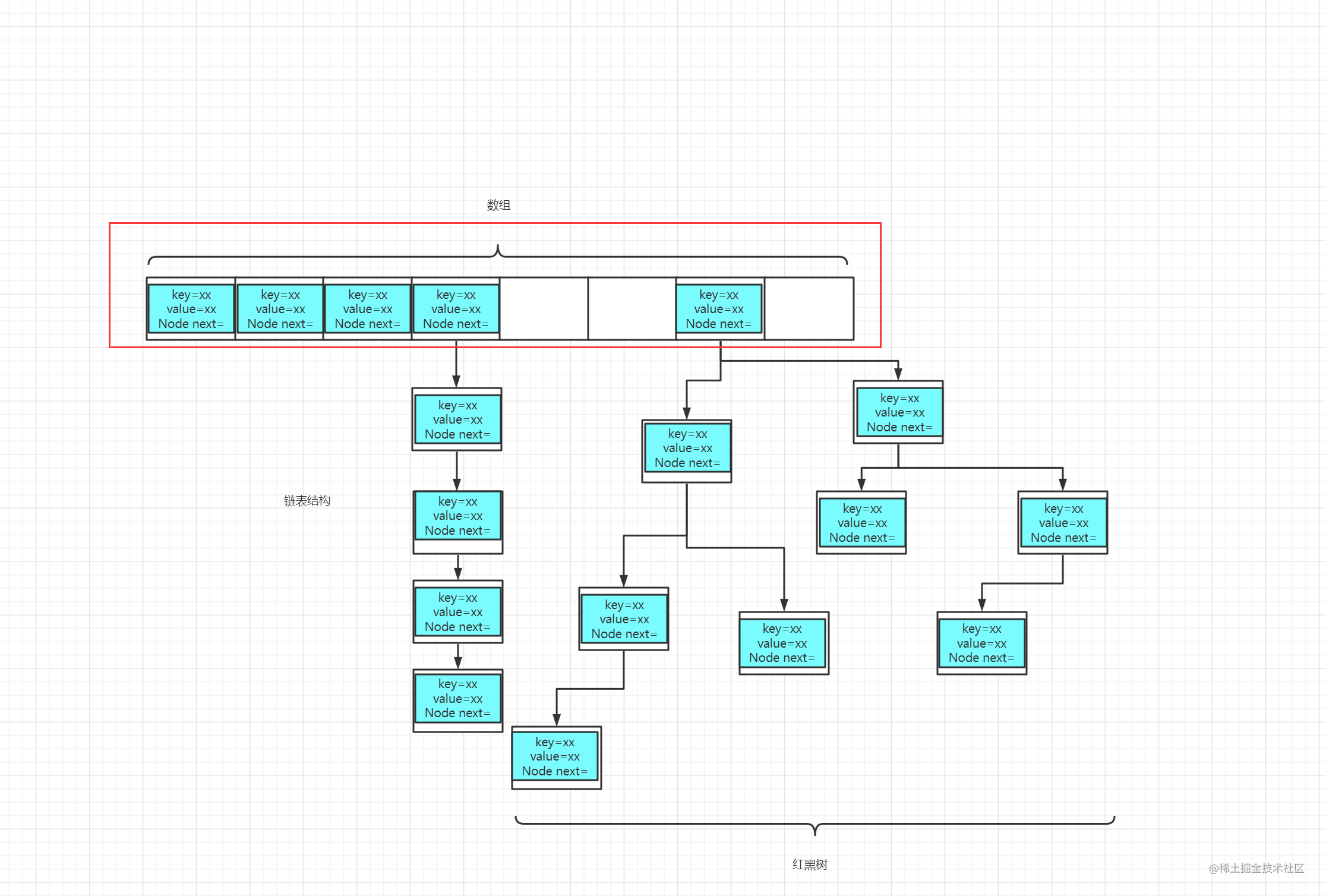• 分析点3：这里就和分析2相反，第一层找不到，那就只有遍历下面对应节点的下一层。如果是链表，那就按链表形式查找；如果是红黑树，那就按照红黑树形式查找；如果找到了，就将对应的节点向上一层返回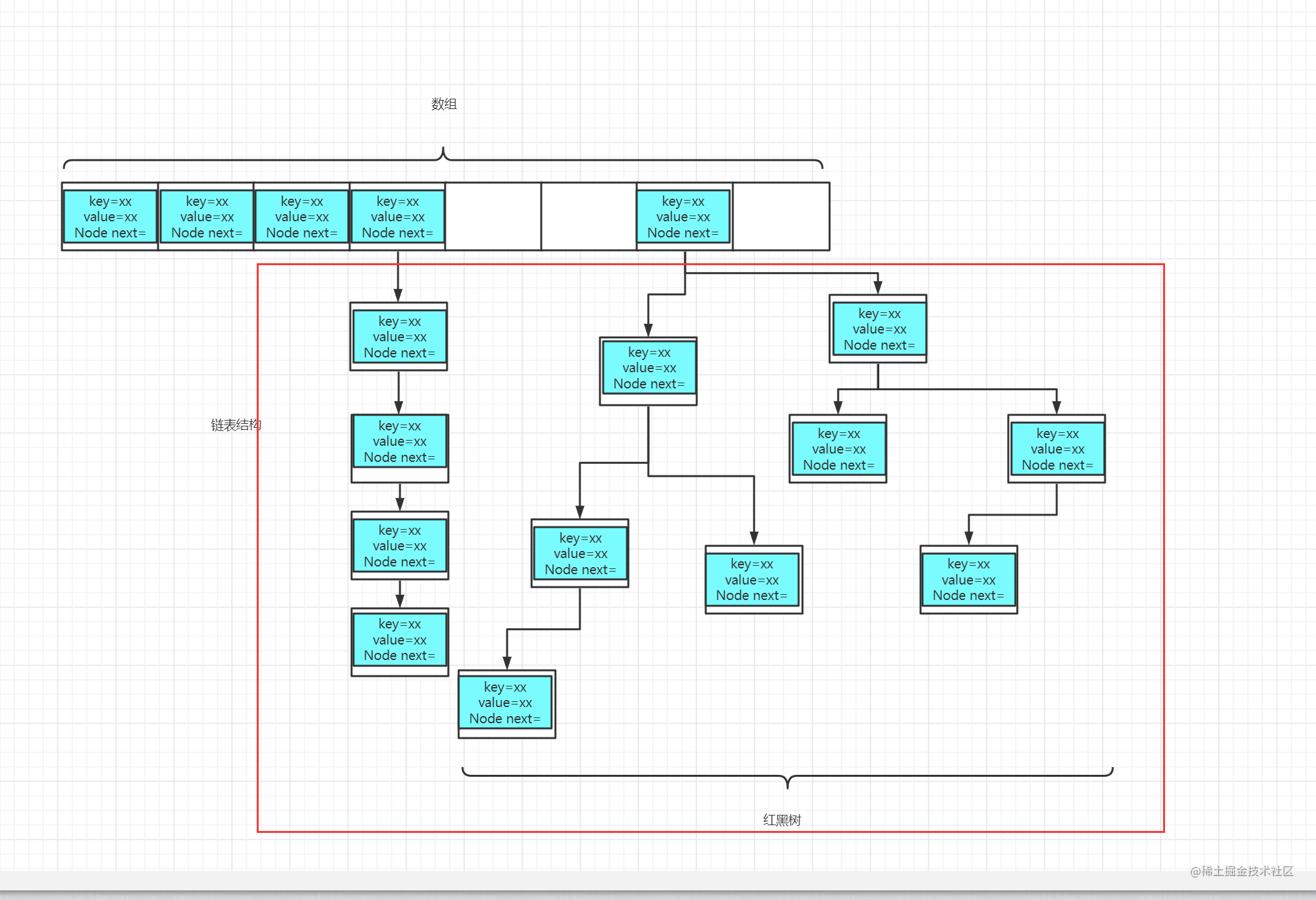• 分析点4：到这里这说明上面所有方式都没有找到对应key相关的节点，因此返回null

## 3、总结

• HashMap的原理？内部数据结构？

• HashMap底层它是有哈希表组成，当链条过长时，将会转化为红黑树结构
• HashMap中put方法的过程是怎样实现的？

1. 对key求hash值，然后再计算下标
2. 如果没有碰撞，直接放入数组中
3. 如果碰撞了，就根据key判断是否存在于链表中，存在则直接覆盖值，不存在则以链表的方式链接到后面
4. 如果链表长度过长（>=8），此时链表将转为红黑树
5. 如果桶满了（容量*加载因子），那么就需要调用resize方法进行扩容
• HashMap中hash函数是怎样实现的？

• 高16bit不变，低16bit和高16bit做了一个异或
• 通过(n-1)&hash 得到对应的下标
• HashMap是怎样扩容的呢？

1. 在resize方法里，首先通过（容量*加载因子）计算出下一次扩容所需要达到的条件
2. 当在putVal，如果对应长度达到了扩容的条件那么就会再次调用resize方法，通过 原长度<<1 移位操作 进行扩容
3. 而对应的扩容条件也会跟随这 原扩容因子<<1 移位操作
• HashMap中某个Entry链太长，查找时间复杂度可能达到O(n)，怎么优化？

• 其实上面已经答了，就是将链表转化为红黑树操作！

Android